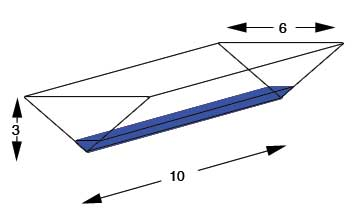SEARCH HOMEMath Central Quandaries & Querieslanny, a student: a triangular trough is 10 feet long, 6 feet across the top, and 3 feet deep. if water flows at the rate of 12 cubic inches per minute, find how fast the surface is rising when the water is 6 inches deep.Hi Lanny,

I assume from the information you gave, the trough looks like this:The volume of the triangular prism is area of the triangle x the length or V=1/2 bhl. Notice that the base of the triangle is twice as big as the height so we could simplify the formula for volume to V=1/2 (2 h)hl=h2l. Also remember that l is constant when the trough is filling so we can substitute 10 feet right into the formula so V=5h2. Lastly, use implicit differentiation to solve for your rate of change but don't forget to make sure all of the units of measurement are the same.

Hope this helps,

JaniceMath Central is supported by the University of Regina and The Pacific Institute for the Mathematical Sciences.